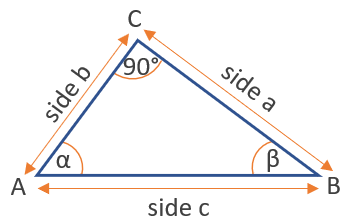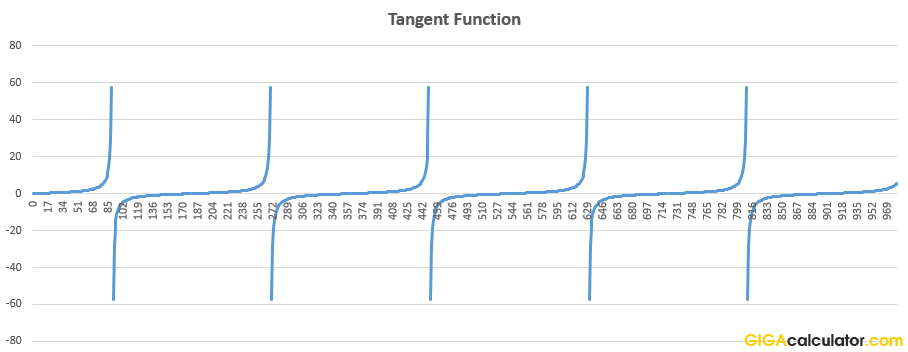# Tangent Calculator

Use this tangent calculator to easily calculate the tangent of an angle given in degrees or radians.

### Calculation results

1 tan(45) =
Share calculator:

Embed this tool:
get code

## Tangent function ( tan(x) )

The tangent is a trigonometric function, defined as the ratio of the length of the side opposite to the angle to the length of the adjacent side, in a right-angled triangle. It is called "tangent" since it can be represented as a line segment tangent to a circle.In the graph above, tan(α) = a/b and tan(β) = b/a. A tangent of an angle α is also equal to the ratio between its sine and cosine, so tanα = sinα / cosα. Following from the definition, the function results in an undefined value at certain angles, like 90°, 270°, 460°, and so on.

## Related trigonometric functions

The reciprocal of tangent is the cotangent: cot(x), sometimes written as cotan(x), which is the ratio of the length of the adjacent side to the length of the side opposite to the angle.

The inverse of the tangent is the arctangent function: arctan(x). It is useful for finding an angle x when tan(x) is known.

## How to calculate the tangent of an angle?

Our tangent calculator accepts input in degrees or radians, so assuming the angle is known, just type it in and press "calculate". Easy as that.

If the angle is unknown, but the lengths of the opposite and adjacent side in a right-angled triangle are known, then the tangent can be calculated from these two measurements. For example, if a = 15 and b = 20, then tan(α) = 15 / 20 = 0.75.

## Applications of the tangent function

The tangent function is used in measuring height of objects located at known distances and has application in flight path and altitude gain calculations. In engineering, it is used to calculate forces of supporting structures, like roof beams. They are also used in robotics to calculate robot arm kinematics.

In an everyday kind of situation, if you are cutting down a tree and want to tether the top to the ground with rope at an X angle, use the tan function to calculate how much rope you would need.Above: the tan calculator output for increasing angle values in degrees.

Table of common tangent values:

Common values of the tangent function
π/6 0
30° π/5 0.577350
45° π/4 1
60° π/3 1.732051
90° π/2 undefined
120° 2π/3 -1.732051
135° 3π/4 0.707107
150° 5π/6 -0.577350
180° π 0

#### Cite this calculator & page

If you'd like to cite this online calculator resource and information as provided on the page, you can use the following citation:
Georgiev G.Z., "Tangent Calculator", [online] Available at: https://www.gigacalculator.com/calculators/tangent-calculator.php URL [Accessed Date: 27 Mar, 2023].